// This prints the left floatting menu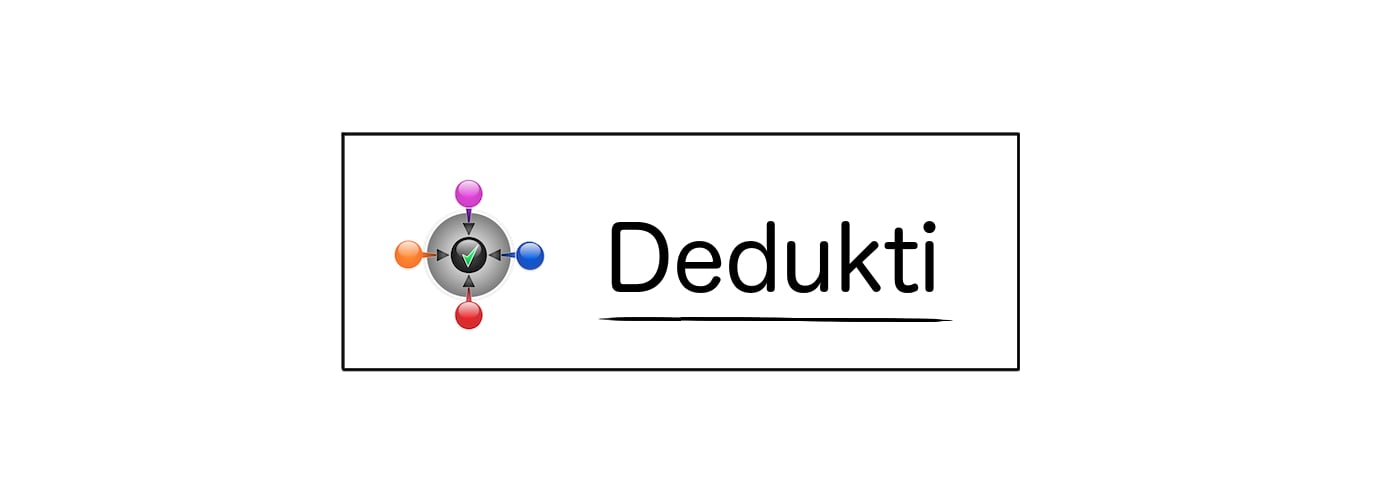### Theoremlogic.eq_rect_r

Statement

∀ a x, x = a ⇒ ∀ P, P a ⇒ P x

Main Dependencies
Theory
constant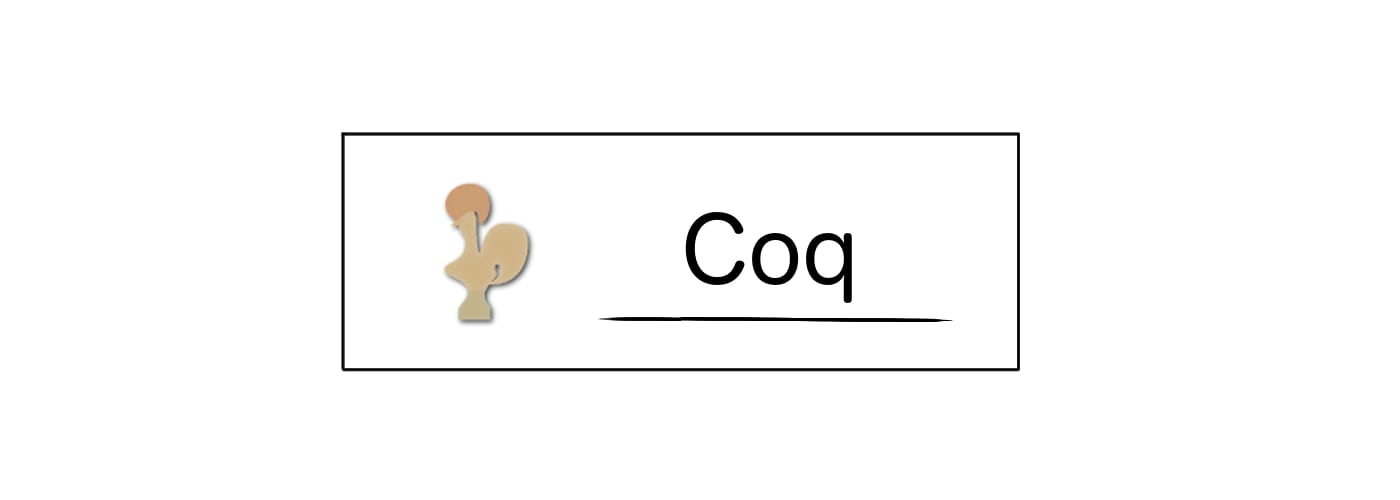Statement

Theorem eq_rect_r : forall A, forall (a:A), forall (x:A), (eq (A) x a) -> forall (P:(A -> Prop)), (P a) -> P x.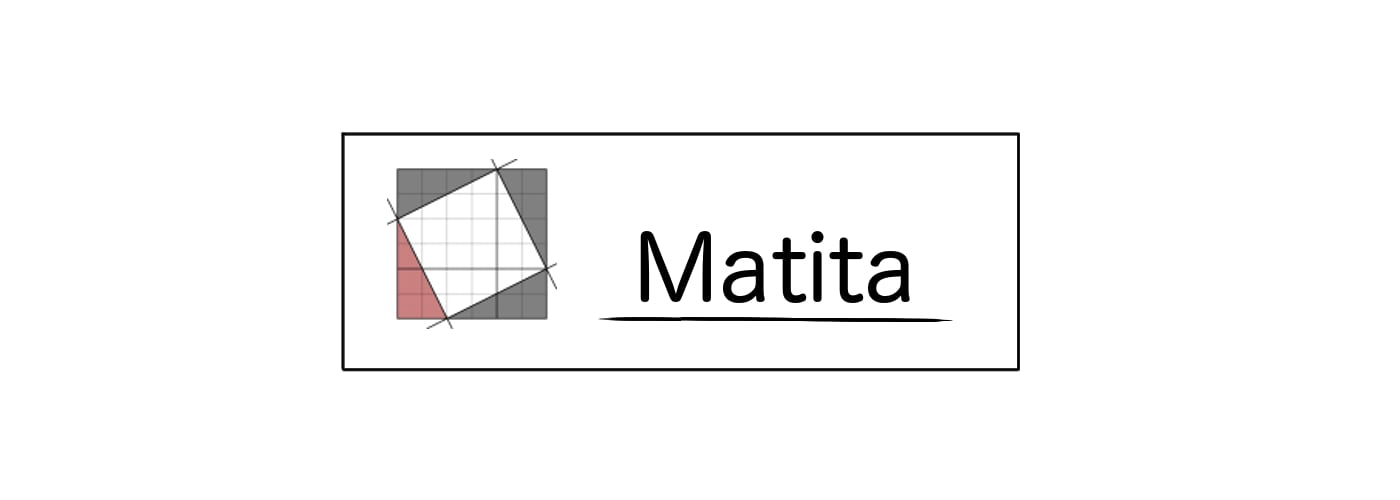Statement

theorem eq_rect_r : \forall A. \forall (a:A). \forall (x:A). ((eq) (A) x a) -> \forall (P:A -> Prop). (P a) -> P x.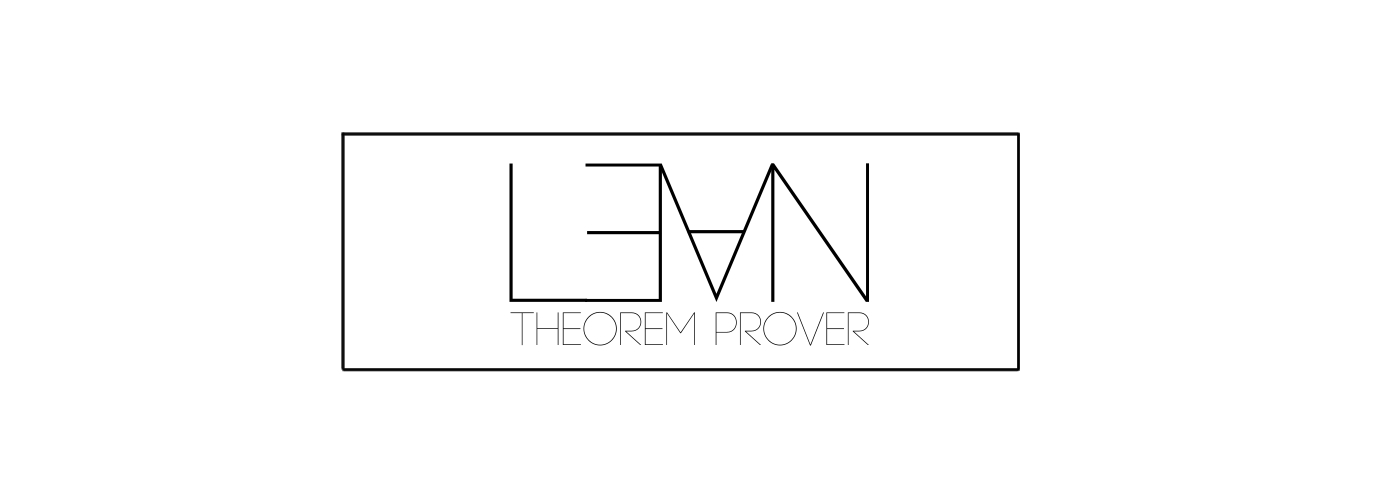Statement

theorem eq_rect_r : forall (A : Type) , forall (a:A) , forall (x:A) , ((((logic.eq_) (A)) (x)) (a)) -> forall (P:(A) -> Prop) , ((P) (a)) -> (P) (x).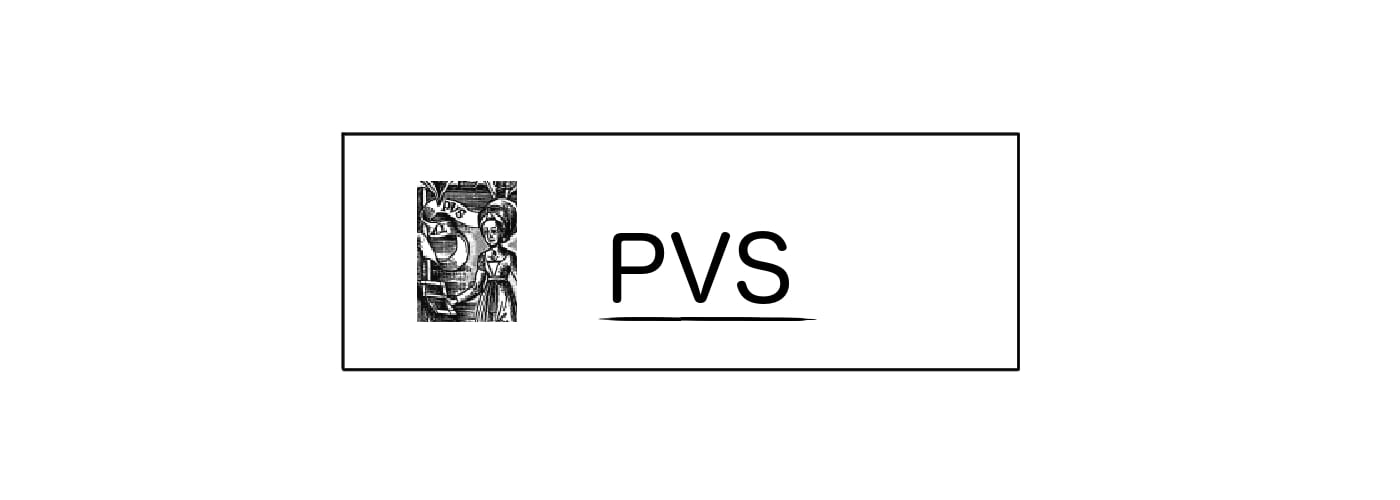Statement

eq_rect_r [A:TYPE+] : LEMMA (FORALL(a:A):(FORALL(x:A):(logic_sttfa.eq[A](x)(a) => (FORALL(P:[A -> bool]):(P(a) => P(x))))))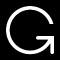Printing for OpenTheory is not working at the moment.